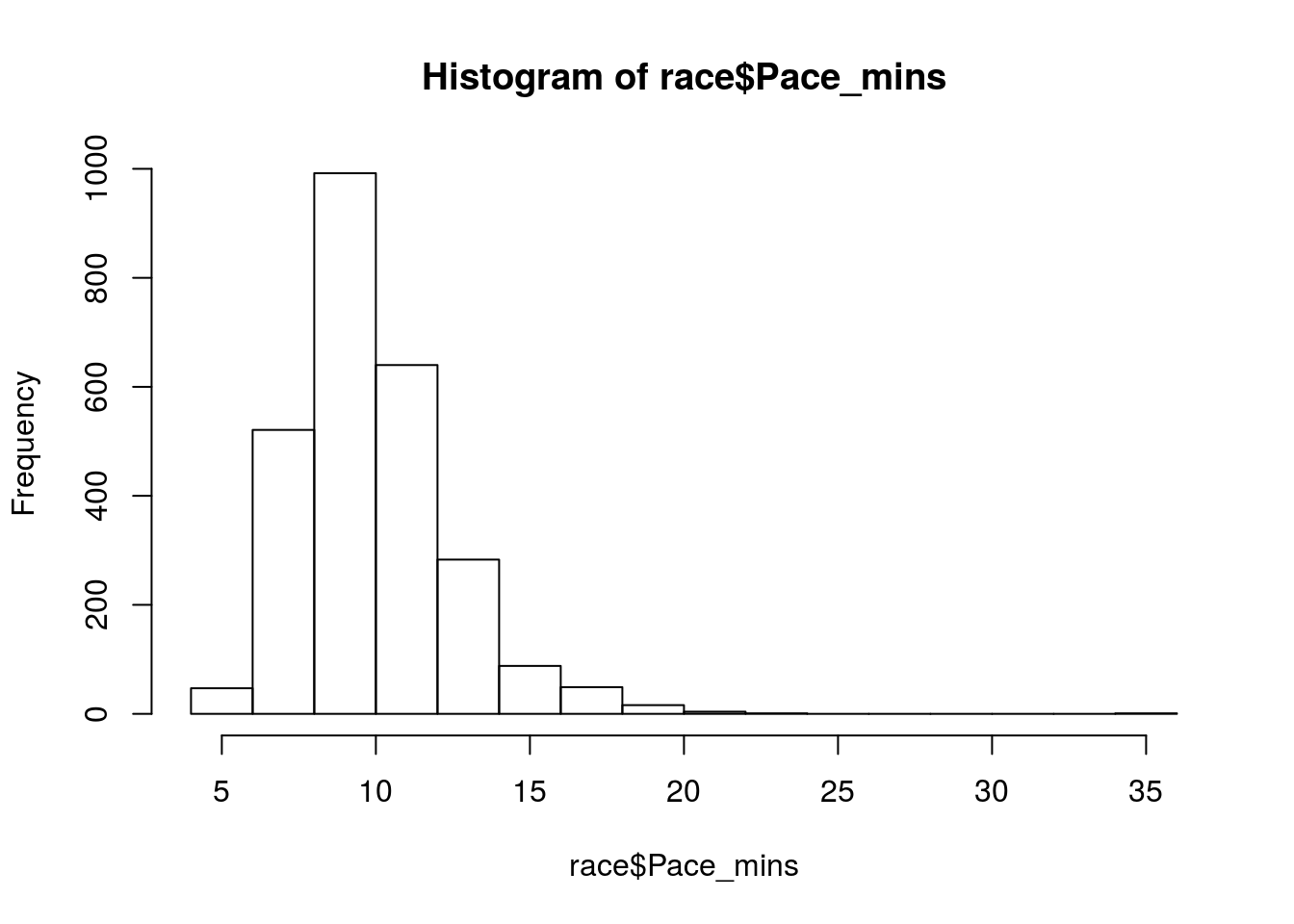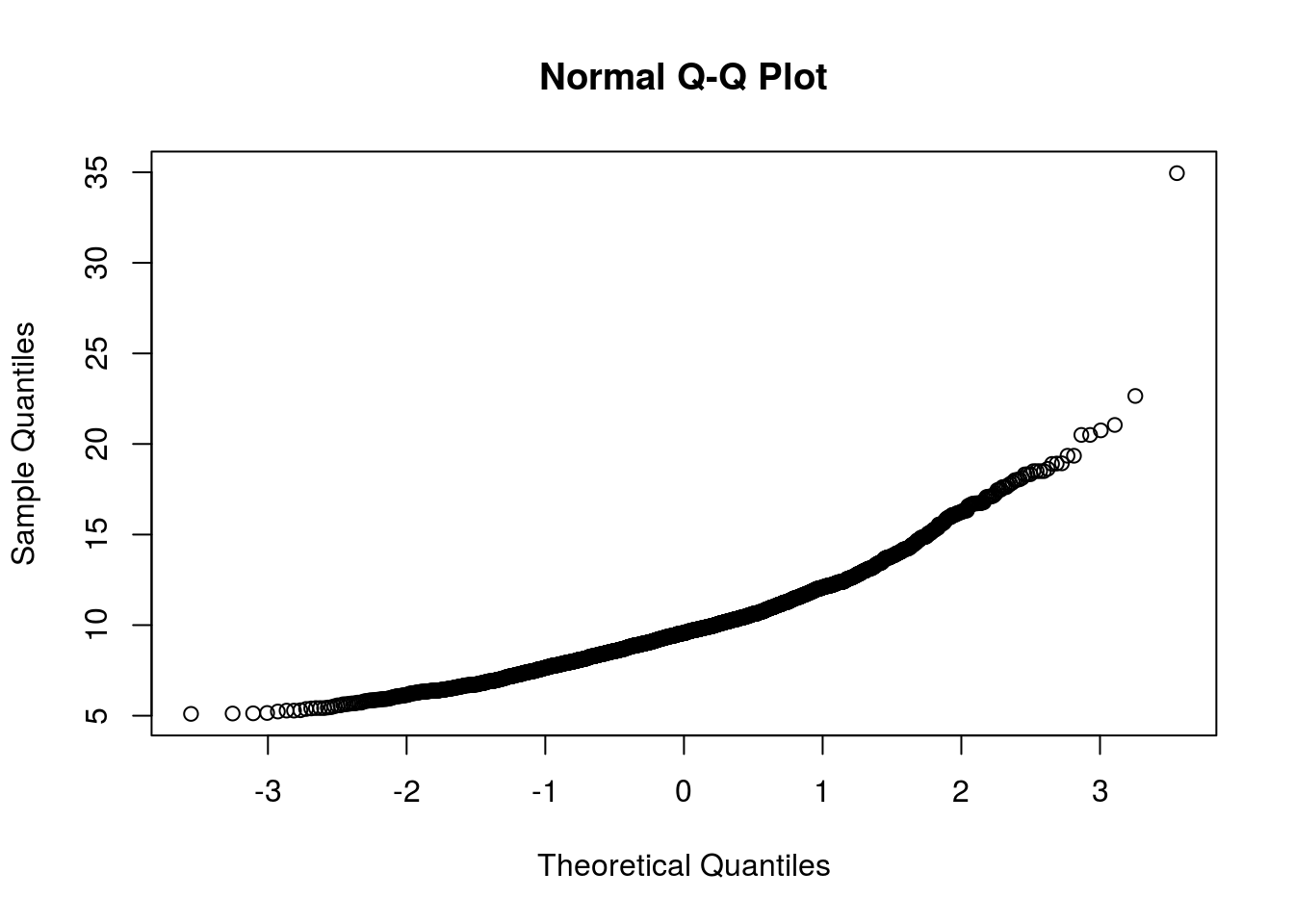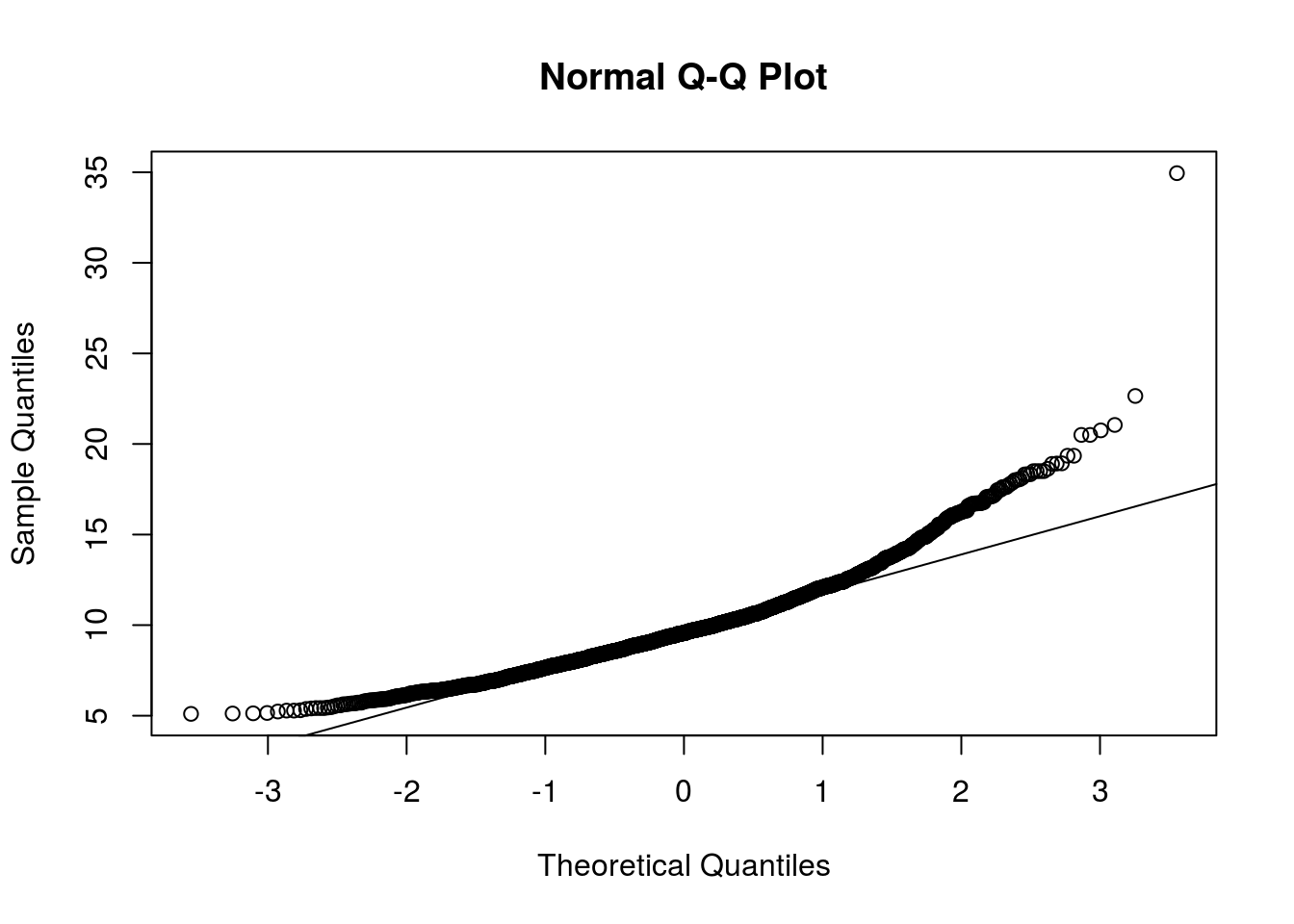Testing_Populations

In this lesson, we will look at methods to test whether samples are drawn from populations with different means, or test whether one sample is drawn from a population with a mean different from some theoretical mean.

We are moving from data that was wholly categorical (i.e., counts of the number of individuals in different groups) in the previous lesson, to comparing continuous data that come from individuals in different groups or categories.

Our overarching question is whether the mean value of individuals in one group is different from the mean value of individuals from another group. Continuing with our previous example, we could ask whether female trees tend to be larger than male trees, on average.

As with the last lesson, we will progress from simple data and analyses to more complex data. First, we will consider comparing the mean of one sample to a known mean. Second, we will compare the means of two independent groups. Third, we will compare the means of paired samples. Finally, we will compare the means of more than two groups.

With all four comparisons, we will illustrate the analysis of parametric data (that follow a Normal, or Gaussian, distribution) and non-parametric (from a non-Normal distribution).

## Data

We will use the data from the 2017 New Haven Road Race. Look at the first few rows of the data, in the `race` object.

``head(race)``
``````##   Place  No.                Name         City Div Sex Class Nettime_mins
## 1     1 4606        Jake Gurzler   Manchester   1   M 30-39        15.82
## 2     2 4384      Patrick Galvin     Stamford   1   M 20-29        15.85
## 3     3 4598       Aidan Pillard   Washington   2   M 20-29        15.92
## 4     4 3745          Omar Perez Poughkeepsie   3   M 20-29        15.98
## 5     5 5807 Timothy Milenkevich      Ansonia   2   M 30-39        16.20
## 6     6 3954     Tim Foldy-Porto  Northampton   1   M 13-19        16.37
##   Time_mins Pace_mins
## 1     15.82      5.10
## 2     15.87      5.12
## 3     15.93      5.13
## 4     16.00      5.15
## 5     16.23      5.23
## 6     16.38      5.28``````

## Testing assumptions: Normality

The t-test and ANOVA assume that the data (or the residuals from the model) follow a Normal distribution. Lets test this assumption visually using a a histogram and/or a quantile-quantile plot, and statistically using a Shapiro-Wilk test (or Kolmogorov-Smirnov test).

Make a histogram of Pace (minutes).

``hist(race\$Pace_mins)``We appear to have a decent right-skew in our data, but lets diagnose using a few other methods.

### The quantile-quantile plot

Plot a q-q plot of the `\$Pace_mins` data (Remember the function `qqnorm()`).

``qqnorm(race\$Pace_mins)``The data look ok, but it can be hard to tell without a reference … let’s add a line to the plot using `qqline()`

``````qqnorm(race\$Pace_mins)
qqline(race\$Pace_mins)``````The data fall away from the line at both ends, suggesting the data are not normally disitributed. D’oh.

### The Shapiro-Wilk test

Check if the `\$Pace` column in the race data is normally distributed using a Shapiro-Wilk test of normality (we would expect not, given that it is a vector of integers from 1 to 2655 giving the final place in the race of each runner).

``shapiro.test(race\$Place)``
``````##
##  Shapiro-Wilk normality test
##
## data:  race\$Place
## W = 0.9549, p-value < 2.2e-16``````

Our data is clearly not normally distributed. This does not inherently mean that we can not use parametric tests for our data - the degree to which our data deviates from normality is more important. We should take caution in interpreting model results however. We will forge ahead anyway.

## A. Compare the mean of one sample to a known mean

### A1. Normal data: the one-sample t-test

First, we can test whether one sample is drawn from a population with a mean different from a known mean, using a one-sample t-test.

This known mean value could come from one of several sources. The mean could come from theory or a theoretical model; it could be data that come from a previous experiment or study; or from an experiment where you have a control and treatment conditions. If you calculate the difference between the treatment and control, you can test whether the mean % difference of the treatment differs significantly from 100.

Similar to the ratio and proportion tests, our null hypothesis would be one of the sample mean is equal to/greater then/less than the theoretical mean.

Let’s test if the mean net time for 2016 was different from the mean net time of 30 minutes and 56 seconds that we have for 2015.

As good data analysts, we should always examine the data before we do anything. Check a summary of the `Nettime_mins` column.

``summary(race\$Nettime_mins)``
``````##    Min. 1st Qu.  Median    Mean 3rd Qu.    Max.
##   15.82   25.23   29.18   30.22   33.85  107.33``````

And now we should plot the data, again, to look at the distribution and to check for odd data points and outliers. We have continuous data from a group, thus a boxplot is probably the best kind of plot. Using the `boxplot() function, make a boxplot of the`Nettime_mins` column.

``boxplot(race\$Nettime``